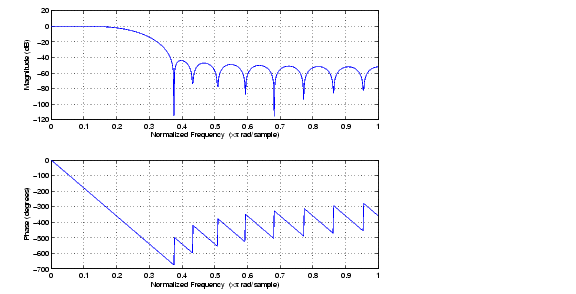Signal Processing Toolboxfirrcos

Raised cosine FIR filter design

Syntax

• ```b` = `firrcos(n,F0,df,fs)
`b = firrcos(n,F0,df,fs,'``bandwidth`'```)
```b` = `firrcos(n,F0,df)
b` = `firrcos(n,F0,r,fs,'`rolloff`')
b` = `firrcos(...,'`type`')
b` = `firrcos(...,'`type`',delay)
b` = `firrcos(...,'`type`',delay,window)
[b,a]` = `firrcos(...)
```

Description

```b = firrcos(n,F0,df,fs) ``` or, equivalently,

```b = firrcos(n,F0,df,fs,'bandwidth') ``` returns an order `n` lowpass linear-phase FIR filter with a raised cosine transition band. The filter has cutoff frequency `F0`, transition bandwidth `df`, and sampling frequency `fs`, all in hertz. `df` must be small enough so that `F0` ± `df/2` is between `0` and `fs/2`. The coefficients in `b` are normalized so that the nominal passband gain is always equal to 1. Specify `fs` as the empty vector `[]` to use the default value `fs` = `2`.

```b = firrcos(n,F0,df) ``` uses a default sampling frequency of `fs` = `2`.

```b = firrcos(n,F0,r,fs,'rolloff') ``` interprets the third argument, `r`, as the rolloff factor instead of the transition bandwidth, `df`. `r` must be in the range `[0,1]`.

```b = firrcos(...,'type') ``` designs either a normal raised cosine filter or a square root raised cosine filter according to how you specify of the string `'``type``'`. Specify `'``type``'` as:

• `'normal'`, for a regular raised cosine filter. This is the default, and is also in effect when the '`type`' argument is left empty, `[]`.
• `'sqrt'`, for a square root raised cosine filter.

```b = firrcos(...,'type',delay) ``` specifies an integer delay in the range `[0,n+1]`. The default is `n/2` for even `n` and `(n+1)/2` for odd `n`.

```b = firrcos(...,'type',delay,window) ``` applies a length `n`+1 window to the designed filter to reduce the ripple in the frequency response. `window` must be a length `n`+1 column vector. If no window is specified, a rectangular (`rectwin`) window is used. Care must be exercised when using a window with a delay other than the default.

```[b,a] = firrcos(...) ``` always returns `a = 1`.

Examples

Design an order 20 raised cosine FIR filter with cutoff frequency 0.25 of the Nyquist frequency and a transition bandwidth of 0.25:

• ```h` = `firrcos(20,0.25,0.25);
freqz(h,1)```

See Also

`fir1`, `fir2`, `firls`, `firpm`firpmord flattopwin© 1994-2005 The MathWorks, Inc.